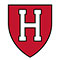Understanding Ridge Regression Using Python

# Understanding Ridge Regression Using Python

4 mins read909 Views 1 Comment
clickHere
Updated on Jan 20, 2023 14:09 IST

Ridge regression is a regularization technique that penalizes the size of the regression coefficient based on the l2 norm. It is mainly used to eliminate multicollinearity in the model. This article will briefly cover all about ridge regression and how to implement in python.

In the previous article, we discussed one of the regularization techniques, Lasso Regression. This article will discuss another regularization technique known as Ridge Regression. Ridge regression is mainly used to analyze multiple regressions that have multicollinearity.

The only difference between the regularization technique is their penalty term; unlike the lasso regression, it uses the square of the coefficient as a penalty term. It is also referred to as L2 regularization.

Before starting the article, lets discuss multicollinearity and its consequences:

In any dataset, multicollinearity occurs when two or more predictors in one regression model are highly correlated.

• Multicollinearity occurs in every dataset but extreme or high multicollinearity occurs whenever two or more independent variables are highly correlated.
• Consequences of Extreme multicollinearity:
• An increase in Standard Error and decrease in value of t-test that leads to acceptance of Null Hypothesis, which should be rejected.
• Increases the value of R-squared, which will affect the model goodness of fit.

Now, without any, let’s dive deep to learn more about ridge regression and how to implement it in python.

## What is Ridge Regression?

Ridge regression is a regularization technique that penalizes the size of the regression coefficient based on the l2 norm.

• It is also known as L2 regularization.
• It is used to eliminate multicollinearity in models.
• Suitable for the dataset that has a higher number of predictor variables than the number of observations.
• It is essentially used to analyze multicollinearity in multiple regression data.
• To deal with the multicollinearity in the dataset, it reduces the standard error by introducing a degree of bias to the regression estimate.
• It reduces model complexity by coefficient shrinkage.
• Ridge regression constraint variable forms a circular shape when plotted.
• The two main drawbacks of Ridge regression are that it includes all the predictors in the final model and is incapable of feature selection.

## Implementation of Ridge Regression in Python

The data was extracted from the 1974 Motor Trend US magazine and comprises fuel consumption and 10 aspects of automobile design and performance for 32 automobiles (1973–74 models). The data set is used to implement Ridge Regression to improve the r2 score of the model.

A data frame with 32 observations on 11 (numeric) variables and 1 categorical variable.

mpg: Miles/(US) gallon

cyl: Number of cylinders

disp: Displacement (cu.in.)

hp: Gross horsepower

drat: Rear axle ratio

wt: Weight (1000 lbs)

qsec: 1/4 mile time

vs: Engine (0 = V-shaped, 1 = straight)

am: Transmission (0 = automatic, 1 = manual)

gear: Number of forward gears

Step – 1: Import Dataset

` `
```#import Libraries import pandas as pdimport numpy as np #import the dataset mt =pd.read_csv('mtcars.csv') #check the first five rows of the data mt.head()Copy code```

Step – 2: Check for Null Values

` `
```#check for null values mt.info()Copy code```

In the above dataset, no feature contains the NULL values.

Step-3: Drop the Categorical Variable

` `
```#drop the categorical variable: model from the dataet mt.drop(['model'], axis = 1, inplace = True)mtCopy code```

Step -4: Create Feature and Target Variable

` `
```#create feature and Target Variable x = mt.drop(columns = 'hp', axis = 1)y = mt['hp']Copy code```

Step- 5: Train- Test Split

` `
```#splitting the data into train and test set from sklearn.model_selection import train_test_split x_train, x_test, y_train, y_test = train_test_split(x, y, test_size = 0.3, random_state = 4)Copy code```

Step – 6: Fitting into the model

` `
```#fitting data into the model from sklearn.linear_model import LinearRegression lr = LinearRegression()lr.fit(x_train, y_train)Copy code```

Step – 7: Checking r-squared value

` `
```#import r2_score from sklearn.metrics import r2_scoreCopy code```
` `
```#r2-score for train data x_pred_tarin = lr.predict(x_train)r2_score(y_train, x_pred_train)Copy code```
` `
```#r2-score for test data x_pred_test = lr.predict(x_test)r2_score(y_test, x_pred_test)Copy code```

Step – 8: Building Ridge Model

` `
```from sklearn.linear_model import Ridge ridge = Ridge()ridge.fit(x_train, y_train)Copy code```
` `
`x_pred_ridge_test = ridge.predict(x_test)r2_score(y_test, x_pred_ridge_test)Copy code`

From above, we get the r2_score increases after implementing ridge changes from 0.54 to 0.80.

## Conclusion

Ridge regression is a regularization technique that penalizes the size of the regression coefficient based on the l2 norm. It is mainly used to eliminate multicollinearity in the model. This article briefly discussed all about ridge regression and how to implement in python.

Top Trending Article

Interview Questions

clickHere

Vikram has a Postgraduate degree in Applied Mathematics, with a keen interest in Data Science and Machine Learning. He has experience of 2+ years in content creation in Mathematics, Statistics, Data Science, and Mac... Read Full Bio

(1)

Dear Vikram, Thank you for this detailed explanation. Please how do I extract the coefficients from this Ridge model?

## Trending Data Science CoursesMachine Learning
Coursera4.6AI for Medicine Specialization
Coursera4.0Starts today

## Top Picks & New Arrivals